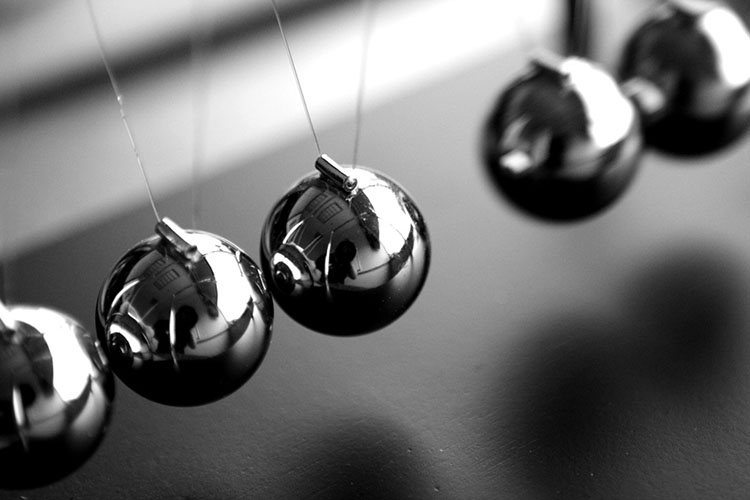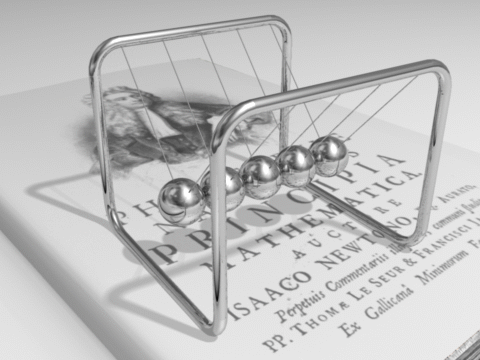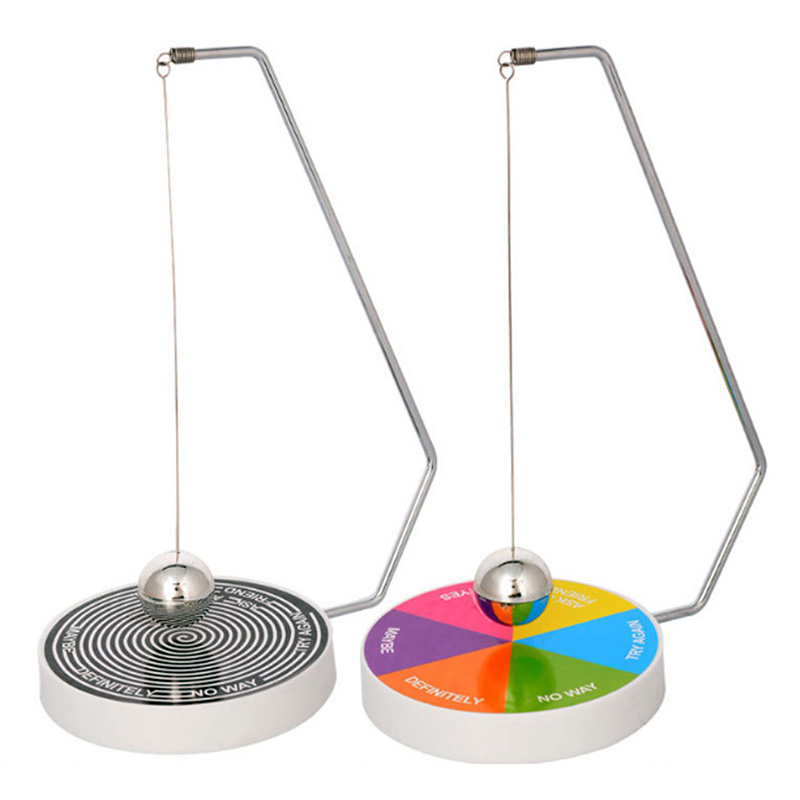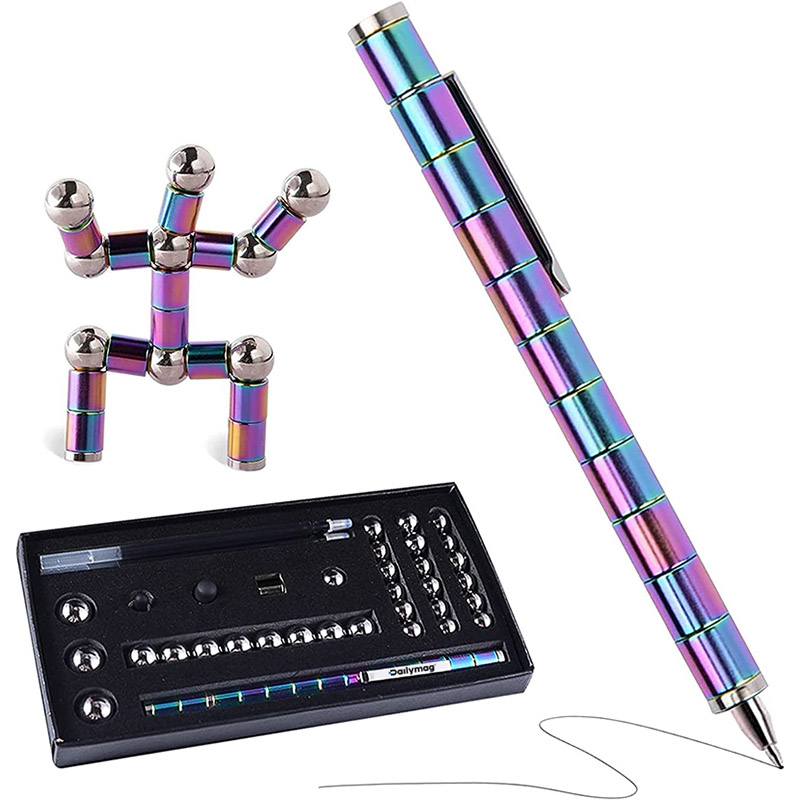### SpecificationsWhat is Newton Cradle, Newton's Cradle, Newton Pendulum, Newton's Pendulum, Newton Balls?

Newton's Cradle is a clever device that not only demonstrates a pendulum, but also shows the Laws of Conservation of Momentum and Energy. Friction and damping effects are also observed. This device is often used as a toy to amuse people.

We are specialized in producing Newton's Cradle.

Newton's Cradle is an excellent choice as a business gift and a good decoration on your desktop. Newton's Cradle is also a good promotional item for you to choose.

Various designs, sizes and colors of Newton's Cradle are available. And client's logos are welcomed.Newton's pendulum was first proposed in 1676 by the French physicist Edme Mariotte. When swinging the rightmost ball and colliding with four other balls in close proximity on the swing back, the leftmost ball will be ejected, and only the leftmost ball will be ejected. Of course, this process is also reversible. When the swinging leftmost ball hits other balls, the rightmost ball will be ejected. When the two balls on the far right swing and hit the other balls at the same time, the two balls on the far left are ejected. The same is true for the opposite direction, and for more balls, three, four, five... .

Ideal situation
There is a problem with the actual Newton's pendulum: a moving ball behaves as if its mass were concentrated in its geometric center. In an ideal Newton's pendulum, the metal spheres are exactly the same mass and will collide perfectly.

Conservation of momentum
The law of conservation of momentum states that in a closed system, the momentum in a given direction is constant. Momentum is expressed as: p=mv (p stands for momentum, m stands for mass, v stands for velocity in a given direction) When ball A hits ball B, it moves in a specific direction, such as from east to west. That means, its momentum (momentum is a vector) also moves in the direction from east to west. Any change in the direction of the ball's motion will result in a change in momentum, which can only be achieved if an external force acts on it. That's why ball A doesn't simply bounce off ball B - its momentum transfers energy through all the balls in an east-to-west direction. In fact, Newton's pendulum is not a closed system, and the metal ball is still under the action of gravity, which will slow down the speed of the small ball until it stops (the momentum is not conserved at this time). When the last ball can no longer transmit momentum and energy, it is bounced off. When it moves to its highest point, it contains only potential energy, and the kinetic energy decreases to zero, gravity moves it downward, and the cycle begins again.

Conservation of energy
The law of conservation of energy states that in a closed system, the total energy is constant. Energy is expressed as representing kinetic energy) When a ball at one end collides with a set of balls with a certain amount of energy, its energy will be transferred to the ball at the other end (instead of disappearing).

Elastic Collision and Friction
An elastic collision occurs when two metal balls collide. The kinetic energy remains unchanged before and after the collision. Under ideal conditions, where the ball is only affected by momentum, energy and gravity, all collisions are perfectly elastic collisions and the structure of Newton's pendulum is perfect, the metal ball will move forever. But a perfect Newton's pendulum can't exist, because it always suffers from friction and energy is lost. Part of the friction comes from air resistance, and most of it comes from the ball itself. So the collision in Newton's pendulum is not a true elastic collision but an inelastic collision, because the kinetic energy after the collision is less than before the collision (due to friction). But according to the law of conservation of energy, the total energy remains the same. Due to the deformation of the ball, the molecules that make up the ball convert kinetic energy into thermal energy. The ball vibrated, producing the crisp crashing sound that is characteristic of Newton's pendulum.

## Related Products##### Magnetic Decision Maker

IQMAG: Magnetic Decision Maker, Executive Decision Maker.##### QQMAG Neocube Magnetic Ball Buckyball Toy

QQMAG (Neocube Magnetic Balls Buckyball) is consisted of super strong NdFeB magnets. ...##### Magnetic Fidget Pen

Magnetic Fidget Pen is a amazing pen that can be a magnetic ...

## GET IN TOUCH

### Send Us a Message

Our experienced and professional team is skilled in designing Magnetic Toy just for you, and we understand the needs of the recycling industry.